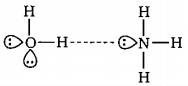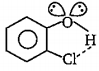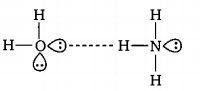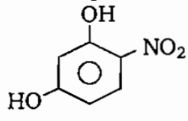The linear structure is possessed by :

(a) $SnC{l}_{2}$

(b) $NC{O}^{-}$

(c) $N{{O}_{2}}^{+}$

(d) $C{S}_{{}^{2}}$

Concept Questions :-

Hybridisation
High Yielding Test Series + Question Bank - NEET 2020

Difficulty Level:

Cis-butene dioic acid $\stackrel{{\mathrm{K}}_{{\mathrm{a}}_{1}}\left\{-{\mathrm{H}}^{+}\right\}}{⇌}{{\mathrm{X}}^{-}}_{1}\stackrel{{\mathrm{K}}_{{\mathrm{a}}_{2}}\left\{-{\mathrm{H}}^{+}\right\}}{⇌}{\mathrm{X}}_{2}^{2-}$

Trans-butene dioic acid $\stackrel{{\mathrm{K}}_{{\mathrm{a}}_{1}}\text{'}\left\{-{\mathrm{H}}^{+}\right\}}{⇌}{{\mathrm{Y}}^{-}}_{1}\stackrel{{\mathrm{K}}_{{\mathrm{a}}_{2}}·\left\{-{\mathrm{H}}^{+}\right\}}{⇌}{\mathrm{Y}}_{2}^{2-}$

The incorrect statement regarding above the information is:

(a) ${\mathrm{X}}_{2}^{2-}$ species is more basic than ${\mathrm{Y}}_{2}^{2-}$ species

(b) ${\mathrm{X}}_{1}^{-}$ species is more basic than ${\mathrm{Y}}_{1}^{-}$ species

(c) ${\mathrm{K}}_{\mathrm{a}1}$ is greater than $\mathrm{K}{\text{'}}_{\mathrm{a}2}$

(d) $\mathrm{K}{\text{'}}_{\mathrm{a}2}$ is greater than $\mathrm{K}{\text{'}}_{\mathrm{a}2}$

Concept Questions :-

Acid, Base and Fajan's Rule
High Yielding Test Series + Question Bank - NEET 2020

Difficulty Level:

In which of the following compounds octet is complete and incomplete for all atoms:

(a)                  IC               IC             IC                     C                         C

(b)                  C                IC             IC                     C                         IC

(c)                  C                IC             C                      IC                        IC

(d)                 IC                C              IC                    IC                        IC

where C denotes Complete and IC incomplete.

Concept Questions :-

Covalent Bonding
High Yielding Test Series + Question Bank - NEET 2020

Difficulty Level:

In which of the following metal of metal bond is present?

(a) Cupric chloride            (b) stannous chloride

(c) mercurous chloride      (d) mercuric chloride

Concept Questions :-

Types of Bonding
High Yielding Test Series + Question Bank - NEET 2020

Difficulty Level:

Which of the following is not best representation of the H-bond?

(a)(b)(c)(d) None of these

Concept Questions :-

Hydrogen Bonding
High Yielding Test Series + Question Bank - NEET 2020

Difficulty Level:

The pair of elemnts which on combination are most likely to form an ionic compound is :

(a) Na and Ca

(b) K and ${O}_{2}$

(c) ${O}_{2}$ and $C{l}_{2}$

(d)

Concept Questions :-

Ionic Bonding
High Yielding Test Series + Question Bank - NEET 2020

Difficulty Level:

The type of molecular forces of attraction present in the following compound is:(a) Intermolecular H-bonding                   (b) Intramolecular H-bonding

(c) van der Waal's force                           (d) All of these

Concept Questions :-

Hydrogen Bonding
High Yielding Test Series + Question Bank - NEET 2020

Difficulty Level:

The species showing $p\pi$-$d\pi$  overlapping is :

(a) $N{{O}_{3}}^{-}$

(b) $P{{O}_{4}}^{3-}$

(c) $C{{O}_{3}}^{2-}$

(d) $N{{O}_{2}}^{-}$

Concept Questions :-

Covalent Bonding
High Yielding Test Series + Question Bank - NEET 2020

Difficulty Level:

Which of the following interaction lies in the range of 8 -  42 kJ/mol?

1. ${\mathrm{H}}_{2}...{\mathrm{H}}_{2}\mathrm{O}$

2. HCl...HCI

3. ${\mathrm{H}}_{2}\mathrm{O}$...HF

4. all

Concept Questions :-

Hydrogen Bonding
High Yielding Test Series + Question Bank - NEET 2020

Difficulty Level:

The number of antibonding electrons pairs in ${{O}_{2}}^{2-}$ on the basis of MO theory are:

(a) 4

(b) 3

(c) 2

(d) 5

Concept Questions :-

M.O.T